# Overview Numeric Attributes for Reporting

Setting up Numerical Attributes.

## Overview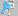Numerical Attributes allow you to create and assign 'quantifying variables' to your functional size measures so you can report a result using Function Points as the other quantifying variable for Project Management Reporting.

SCOPE takes the 'quantifying variable' you set up in the Numerical Category and uses it as a 'multiplier' for the function points it calculates, to create a derived result.

For example if your quantifying variable is Project Delivery rate measured in Units of "hours per fp". Then SCOPE will take the rate and multiply it by Function Points calculated to get "hours" to deliver that many function points.

Since they can be used to report any variable using any unit, then both the variable and the units need to be first to be set up. The setup of the Numerical Category can be completed two ways:

1. Set up Template Numerical Category - for ongoing 're-use' - one set up in the database it is available across all Releases and is automatically loaded into a new Release. It can be also selectively loaded into any Release in the database. see Instructions in Set up Numerical Attributes - (Template).

2. Set up One-off Numerical Category - specific for this Release's Attributes Tree. It can be manually copied and pasted (exported / imported) into another Release tree. See instructions in Set up Numerical Attributes - (non-Template).

## Setting up a Numerical CategoryNumerical Categories are completely customisable, you can create your own, use the built in Numerical Template Categories. Once inserted they can be modified to suit your needs.

In the screen display below you can see how the Name, Units and Variable text is inserted into the reported result to provide meaning to the answer

Variable: this is the scaling factor you want to apply to the Function points to get a result. e.g. project delivery rate = PDR hrs/fp. The individual Values (e.g. Java = 9 hrs /fp) for this scaling factor are recorded in the Attribute details screen.

Units: these are units reported for the result of the multiplication.

For example if the Variable =

• PDR (hours per function point) then the units for the result after multiplying by function points will be 'hours'
• Cost Effectiveness (\$/function point) then the units for the result after multiplying by function points will be 'dollars'
• Worked FPs (percentage) then the units for the result after multiplying by function points will be 'function points'

Once you have set up a Numerical Category, you will need to set up the Numerical Attributes with the relevant Values to be used as the multiplying scaling factor.

Example 1. Setting up a Numerical Category for PDR - On the left side of the Screen is the Details for the Numerical Category. You can see how each of the above fields in the detail screen on the left are reported in the result on the screen on the right.

Units = (Hours) Effort Required

Variable = Project Delivery Rate (PDR)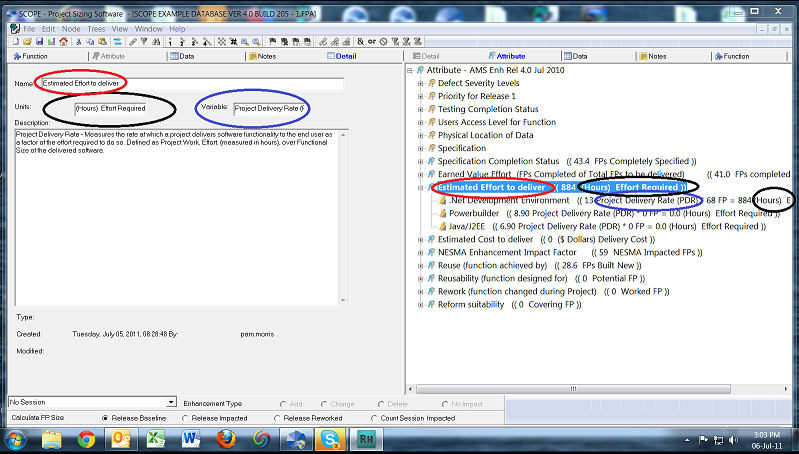## Setting up a Numerical Attribute

Insert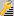Numerical Attributes under the Numerical Category to set up the Values for the possible scaling factors that can be applied to the function points calculated.

For example if the Variable = PDR then we need to set up different PDR Values for different technologies.

Attribute 1 = .Net Development Environment Value = 13.0

Attribute 2 = Powerbuilder Value = 8.9

Attribute 2 = Java/J2EE Value = 6.9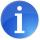Numerical attributes are 'mutually exclusive' ie. a process or a data group can only be linked to ONE numerical attribute within a Numerical Category.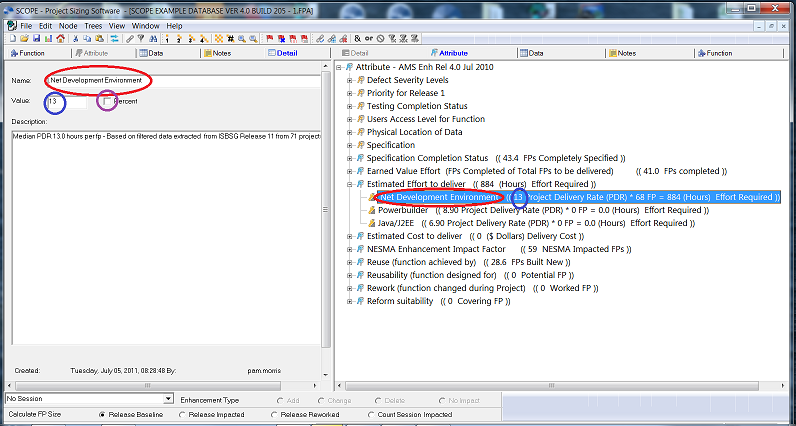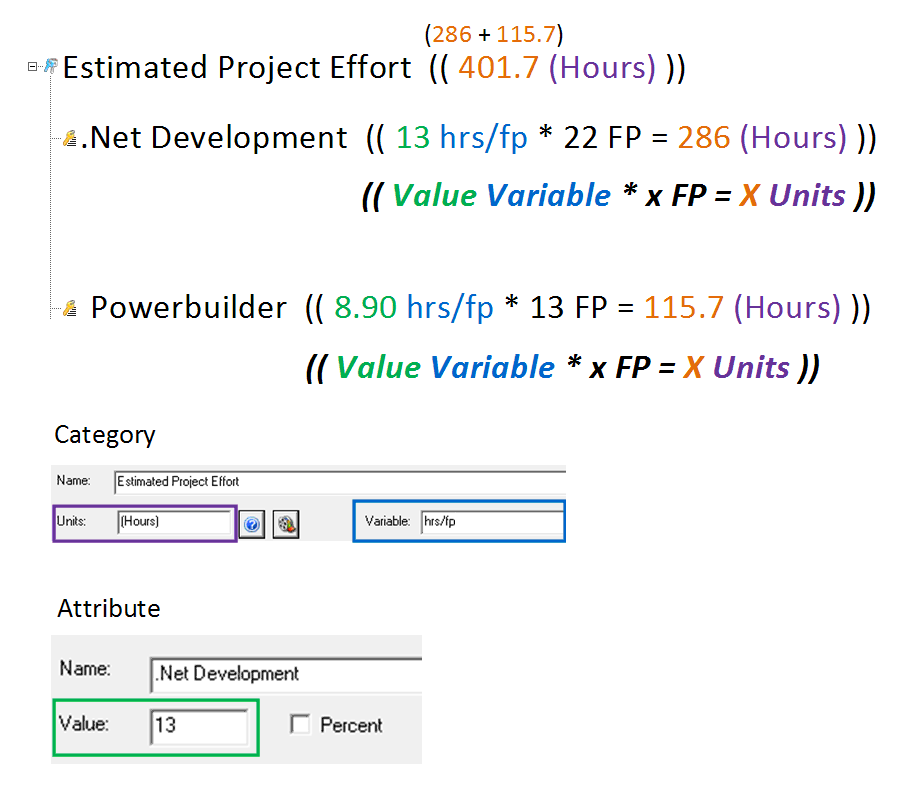For examples on how Numerical Attributes can be used see:

Earned Value Project Monitoring

Estimating Project Effort

Package Implementation Costing